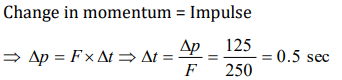## Laws of Motion Questions and Answers Part-14

1. A student attempts to pull himself up by tugging on his hair. He will not succeed
a) As the force exerted is small
b) The frictional force while gripping, is small
c) Newton's law of inertia is not applicable to living beings
d) As the force applied is internal to the system

Explanation: As by an internal force momentum of the system can not be changed.

2. A man is standing at the centre of frictionless pond of ice. How can he get himself to the shore
a) By throwing his shirt in vertically upward direction
b) By spitting horizontally
c) He will wait for the ice to melt in pond
d) Unable to get at the shore

Explanation: By spitting horizontally

3. A lift is going up. The total mass of the lift and the passenger is 1500 kg. The variation in the speed of the lift is as given in the graph. The tension in the rope pulling the lift at t = 11th sec will bea) 17400 N
b) 14700 N
c) 12000 N
d) Zero

Explanation: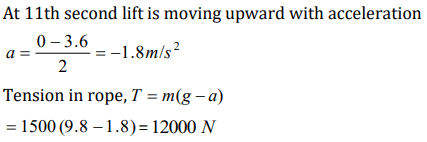4. A jet plane flies in the air because
a) The gravity does not act on bodies moving with high speeds
b) The thrust of the jet compensates for the force of gravity
c) The flow of air around the wings causes an upward force, which compensates for the force of gravity
d) The weight of air whose volume is equal to the volume of the plane is more than the weight of the plane

Explanation: The thrust of the jet compensates for the force of gravity

5. A player caught a cricket ball of mass 150 gm moving at a rate of 20 m/s. If the catching process be completed in 0.1 s, then the force of the blow exerted by the ball on the hands of the player is
a) 0.3 N
b) 30 N
c) 300 N
d) 3000 N

Explanation: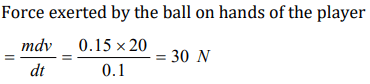6. A rocket has a mass of 100 kg. 90% of this is fuel. It ejects fuel vapours at the rate of 1 kg/sec with a velocity of 500 m/sec relative to the rocket. It is supposed that the rocket is outside the gravitational field. The initial upthrust on the rocket when it just starts moving upwards is
a) Zero
b) 500 N
c) 1000 N
d) 2000 N

Explanation: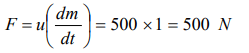7. In which of the following cases forces may not be required to keep the
a) Particle going in a circle
b) Particle going along a straight line
c) The momentum of the particle constant
d) Acceleration of the particle constant

Explanation:8. A wagon weighing 1000 kg is moving with a velocity 50km/h on smooth horizontal rails. A mass of 250 kg is dropped into it. The velocity with which it moves now is
a) 2.5 km/hour
b) 20 km/hour
c) 40 km/hour
d) 50 km/hour

Explanation: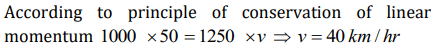9. If a force of 250 N act on body, the momentum acquired is 125 kg-m/s. What is the period for which force acts on the body
a) 0.5 sec
b) 0.2 sec
c) 0.4 sec
d) 0.25 sec